#Application of Fourier Series to Differential EquationsSince the beginning Fourier himself was interested to find a powerful tool to be used in solving differential equations. Therefore, it is of no surprise that we discuss in this page, the application of Fourier series differential equations. We will only discuss the equations of the form

y(n) + an-1y(n-1) + ........+ a1y' + a0 y = f(x),

where f(x) is a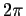-periodic function.

Note that we will need the complex form of Fourier series of a periodic function. Let us define this object first:

Definition. Let f(x) be-periodic. The complex Fourier series of f(x) is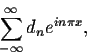where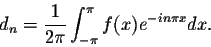We will use the notation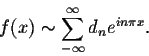If you wonder about the existence of a relationship between the real Fourier coefficients and the complex ones, the next theorem answers that worry.

Theoreme. Let f(x) be-periodic. Consider the real Fourier coefficients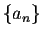and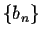of f(x), as well as the complex Fourier coefficients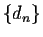. We have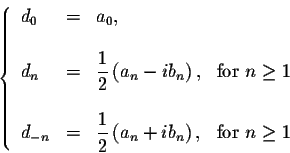The proof is based on Euler's formula for the complex exponential function.

Remark. When f(x) is 2L-periodic, then the complex Fourier series will be defined as before where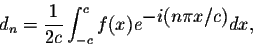for any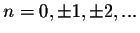.

Example. Let f(x) = x, for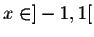and f(x+2) = f(x). Find its complex Fourier coefficients.
Answer. We have d0 = 0 and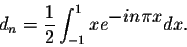Easy calculations give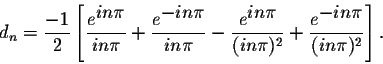Since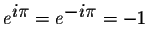, we get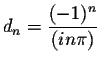. Consequently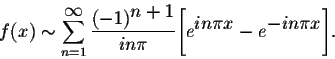Back to our original problem. In order to apply the Fourier technique to differential equations, we will need to have a result linking the complex coefficients of a function with its derivative. We have:

Theorem. Let f(x) be 2L-periodic. Assume that f(x) is differentiable. If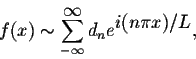then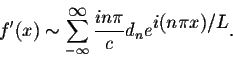Example. Find the periodic solutions of the differential equation

y' + 2y = f(x),

where f(x) is a-periodic function.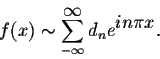Let y be any-periodic solution of the differential equation. Assume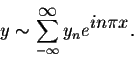Then, from the differential equation, we get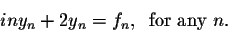Hence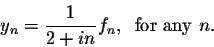Therefore, we have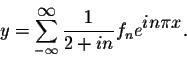Example. Find the periodic solutions of the differential equation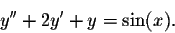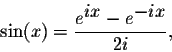we get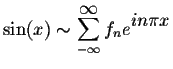with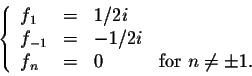Let y be a periodic solution of the differential equation. If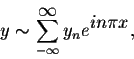then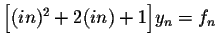. Hence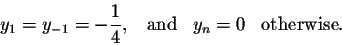Therefore, the differential equation has only one periodic solution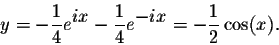The most important result may be stated as:

Theoreme. Consider the differential equation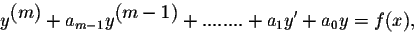where f(x) is a 2c-periodic function. Assume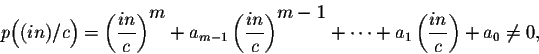for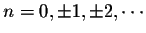. Then the differential equations has one 2c-periodic solution given by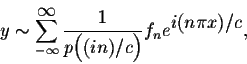where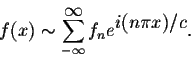[Geometry] [Algebra] [Trigonometry ]
[Calculus] [Differential Equations] [Matrix Algebra]S.O.S MATH: Home Page

Do you need more help? Please post your question on our S.O.S. Mathematics CyberBoard.Author: M.A. Khamsi